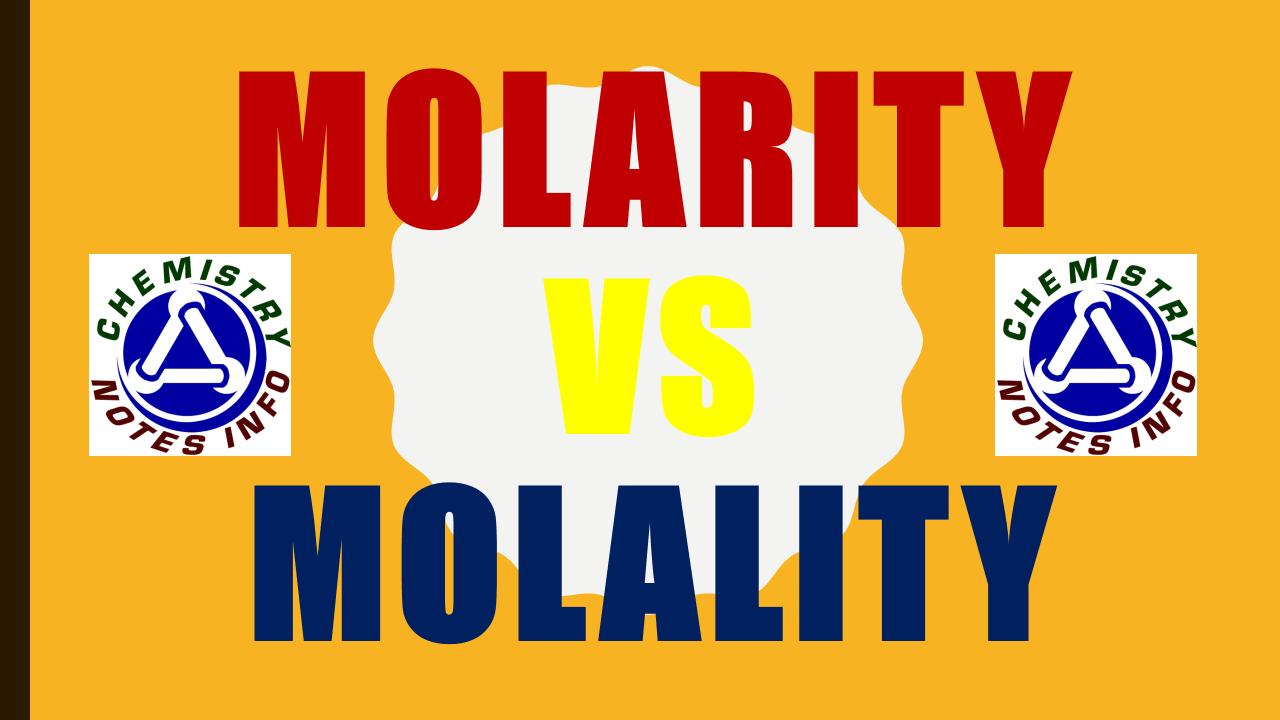# Molarity vs Molality## What is Molarity?

Molarity is also known as molar concentration, it is the ratio of moles of substance to volume in liter.
Where mole is weight in gram divided by molecular weight.
Molarity is chemistry terminology.

### Molarity (M) :-

No. of moles of the solute  / Volume of the solution in liter.
M = strength in gram per liter / Molar mass of the solute
Unit =>   M = Moles/L

## What is Molality?

It is the ratio of moles of substance to kilogram of solvent.
Where mole is weight in gram divided by molecular weight.
Molality is chemistry terminology.

### Molality (m) :-

No. of moles of the solute  Mass of solvent in Kilogram (Kg).

 Molarity vs Molality Chemistry Infographics